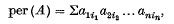Home

Inequalities for permanents and permanental minors

Extract

Let Ωn denote the convex set of all n × n doubly stochastic matrices: chat is, the set of all n × n matrices with non-negative entries and row and column sums 1. If A = (aij) is an arbitrary n × n matrix, then the permanent of A is the scalar valued function of A defined bywhere the subscripts i1, i2, …, in run over all permutations of 1, 2, …, n. The permanent function has been studied extensively of late (see, for example, (1), (2), (3), (4), (6)) and it is known that if A ∈ Ωn then 0 < cn ≤ per (A) ≤ 1, where the constant cn depends only on n. It is natural to inquire if per (A) is a convex function of A for A ∈ Ωn. That this is not the case was shown by a counter-example given by Marcus and quoted by Perfect in her paper ((5)). In this paper, however, she shows that per (½I + ½A) ≤ ½ + ½ per (A) for all A ∈ Ωn. Here I = In is the identity matrix of order n.

Hide All

Metrics

Full text viewsFull text views reflects the number of PDF downloads, PDFs sent to Google Drive, Dropbox and Kindle and HTML full text views.

Total number of HTML views: 0
Total number of PDF views: 0 *Loading metrics...

Abstract viewsAbstract views reflect the number of visits to the article landing page.

Total abstract views: 0 *Loading metrics...

* Views captured on Cambridge Core between <date>. This data will be updated every 24 hours.

Usage data cannot currently be displayed